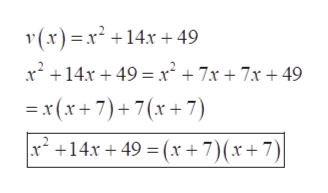# Put the function into factored form with integer coefficients and then identify any horizontal intercepts.v(x)=x2+14x+49Write the points in ascending order of their x-coordinates. If there is only one horizontal intercept, enter the same coordinates for both points.

Question
1 views

Put the function into factored form with integer coefficients and then identify any horizontal intercepts.

v(x)=x2+14x+49

Write the points in ascending order of their x-coordinates. If there is only one horizontal intercept, enter the same coordinates for both points.

check_circle

Step 1

Refer to the question we need to identify the horizontal intercepts by putting the provided function into factorised form.

Step 2

Now to factorise the provided equation we need to split the middle term in such a way that when multiplied it ...help_outlineImage Transcriptionclose(x)x 14x+ 49 x214x49 x* + 7x + 7x+ 49 x(x+7)+7(x +7) +14x 49(x+7)(x+7)| fullscreen

### Want to see the full answer?

See Solution

#### Want to see this answer and more?

Solutions are written by subject experts who are available 24/7. Questions are typically answered within 1 hour.*

See Solution
*Response times may vary by subject and question.
Tagged in

### Polynomials